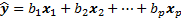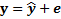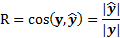Sunday, March 30, 2014

Book review: The Geometry of Multivariate Statistics

This book was written by the late professor Thomas D. Wickends, and is by far the best book on understanding linear regression I have read. As written in the preface, most books on multivariate statistics approach it via two aspects: algebra and computation. Algebraic approach to the multivariate statistics, and multiple regression in particular, is a self-contained one. It is built on linear algebra and probability and therefore can use these two to develop all the theorems and algorithms, and give rigorous proves on the correctness and error bounds of the methods. Computational approach is to teach the students on how to run the software and interpret the results. For example, reading the p-values of the regression coefficients. However the computational approach, because of its lack of diving into the theories, does not tell one how F-test and the corresponding p-values will change when the properties of the data change. And the computational approach would leave statements like “insignificant p-values don’t mean no correlation with the target” at a superficial level without any deep understanding. The geometrical approach taken by Prof. Wickends has the exact aim: to understand the meanings behind the linear algebra equations and the output of the software!

Geometry is a perfect tool for understanding multivariate statistics because first it can help understand linear algebra (quite a few linear algebra books take a geometry approach) and second it can help understand probability distribution as well. For one-dimension distributions, we think in terms of PDF and CDF curves. And for multivariate distributions, the pdf contour in the high dimensional space is elliptical. This book combines these two geometrical understandings in a unified framework and develops several key concepts of multivariate statistics in only 160 pages!

The main feature of this book is that every concept is developed geometrically, without using linear algebra or calculus at all. In viewing the data points, there are two spaces to view them: variable space (row space) and subject space (column space). When the number of variables exceeds three, it becomes hard to think about/visualize the data points in the variable space, yet it is still conceptually very easy to see them in the subject space! And most of the concepts in this book are developed in this subject space, only occasionally the author switches to the variable space to provide another view (e.g. in the PCA chapter). For example, the linear regression equation:(all vectors here are demeaned, so there is no offset parameter a)

Basically this equation defines a linear space that are spanned by x_1 to x_p. The aim of the linear regression is to make \hat{y} as close to the target y as possible. In the subject space:Where e is the residual vector, which is perpendicular to the space defined by xs when the distance (|e|) is minimized. By setting the perpendicular constraints as equations, we can drive the normal equation of linear regression, which in an algebraic approach is usually defined directly as X’X b = X’y rather than derived from geometrical intuition.

Other multivariate statistics book (e.g. Johnson & Wichern) though at places introduce a geometrical explanation. These bits are however embedded inside a large set of linear algebra equations. That makes the geometrical explanation in those book only supportive for the algebra equations, and therefore are not self-contained! While in this book, everything has to be developed geometrically, there is no corner that the author can hide a geometry concept that has not developed before. For example, this book gives a very good explain of the demean operation. In multiple regression, the demean operation introduces a space defined by a 1 vector (1,1,…1)’, and the original y and xs share their mean components in this same space. And this space is perpendicular to the residual vector e, therefore e’1 = 0! (If e’1!=0, then e has a non-zero projection to 1-space, which can then be absorbed into the constant offset in the regression equation.)

For practitioners, section 4.2 (goodness of fit) and chapter 5 of this book are most useful. The coefficient of determination is interpreted geometrically as:Chapter 5’s title is Configurations of multiple regression vectors, which explains how the regression becomes for different configurations of xs. When the predictors (xs) are highly correlated, the regression becomes unstable (more analysis in Chapter 6). And the book proposes several approaches to deal with the multicollinearity problem. One of them is not to minimize |e| alone (as the position of the regression space defined by the collinear xs is not stable), and |e| + some criterion that stabilizes the position of the regression space. This would lead to ridge regression and other variants. Ridge regression is l2-regularized regression, and the regularization is to “control model complexity”. And in the situation of multicollinearity, we can a much better understanding of what on earth is model complexity through geometry!

Other points in this book: The geometry of linear algebra meets the geometry of probability in Chapter 6 (tests). Geometry interpretation of analysis of variance (Sec 3.4 & Chapter 8), PCA (Chapter 9), and CCA (Chapter 10). Each chapter of this book refreshes some of my memory and adds something new, geometrically. Strongly recommended for anyone who works with regression problems and does data mining in general.

This last paragraph is not about this particular book, but is about my motivation of reading statistics books recently. Many data miners understand regression as loss minimization, probably plus knowing that a small p-value is good indication of a useful feature (this is indeed not accurate). I was such a data miner until half a year ago. But few of them know how the p-value in multiple regression (and in other models, e.g. logistic regression) is actually calculated. Software packages nowadays are very advanced and make models like linear regression/pca/cca seem to be as simple as one line of code in R/Matlab. But if we don’t know them by hand and by heart and understand the various properties of these simple models, how can we feel confident when applying them? On the other side, as researchers in data mining, how can we confidently modify existing models to avoid their weakness in a particular application if we only have a shallow understanding of the weakness of a model? Therefore, for example, we cannot stop our understanding of regularization at only one sentence “controlling model complexity.” We need go deeper than that, to know what is behind that abstract term “model complexity”. This is why recently while writing my phd thesis, I am also learning and re-learning statistics and machine learning models. I should have done this say four years ago! But it is never too late to learn, and learning them after doing several successful data mining projects gives me a new perspective.